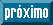## Indicadores

•Citado por SciELO
•Acessos

## Links relacionados

•Similares em SciELO

## versão impressa ISSN 2007-1132

#### Resumo

HERNANDEZ RAMOS, Jonathan et al. Ratio factors and normal diameter equations from the stump diameter of Pinus greggii Engelm. Rev. mex. de cienc. forestales [online]. 2016, vol.7, n.35, pp.7-18. ISSN 2007-1132.

When normal diameter (dn) of trees for volume calculations are unknown, the functional relationship between dn and stump diameter (dt ) is very useful. The aim of this study was to generate equations and dn-dt ratio factors for Pinus greggii plantations in the state of Hidalgo , Mexico. With the data from 621 trees, four models were fitted to estimate the dn-dt relation to the PROC MODEL SAS 9.1 statistical package and the gmm procedure.The best model was selected by the fitted Coefficient of Determination (R2 aj. ), the Root Mean Square Error (REMC), the Akaike (AIC) and Bayesian (BIC) information criteria, the graphical analysis of the residuals and the normality test. Predictability was determined with the bias (E), Aggregate Deviation (DA ) and DA percentage (DA %), in addition to the graphical behavior of the predicted values against the observed ones. The selected equation included stump height (ht ) and explained 94.86 % of the observed variance, without violating the assumptions of normality of the data, residual homoscedasticity and the autocorrelation of errors. For the sample, the bias was -0.0352, -0.0353 for DA and 0.2032 for DA %. The average ratio factor between dt and dn was 0.7879. This equation and the ratio factor are reliable tools for estimating dn, and may be applied as the basis for estimating volume, biomass or carbon in P. greggii plantations.

Palavras-chave : Allometry; logging; tree biomass; illegal cutting; equations; volume.

· resumo em Espanhol     · texto em Espanhol | Inglês     · Inglês (pdf ) | Espanhol (pdf )